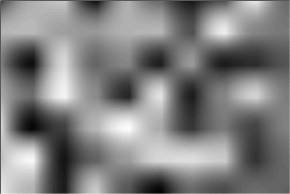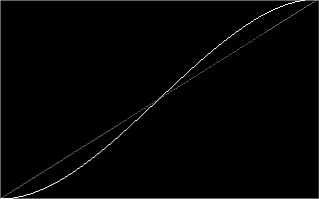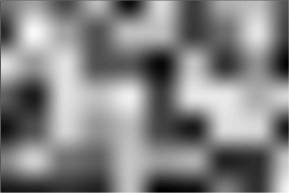# Bilinear filtering tutorial

## Bilinear filtering

Bilinear filtering is an algorithm to interpolate between pixels often used to render textures or pictures in a size larger than the original. Usually a larger version of a picture looks ugly because you can see the rectangle structure of the pixels.

## 1D interpolation

The solution is to interpolate the colors to get a smooth fading from one color to another. An easy 1D interpolation can be done with the following formula:

result = position * col1 + (1 - position) * col2

where col1 is the first color, col2 is the second color and position is a number between 0 and 1 that determines the position between this colors. If you fade two colors with this formula, you get a smooth looking result (for RGB colors, you just have to fade each component seperate).

## 2D interpolation

When you fade 2D data it is getting harder, because you have to fade between 4 pixel colors. But you can still use the formula above. First fade between the two colors on the edges above and below and then fade between these two colors:

col5 = posx * col1 + (1 - posx) * col2
col6 = posx * col3 + (1 - posx) * col4

result = posy * col5 + (1 - posy) * col6

where col1 - col4 are the original four colors, col5 and col6 are the interpolations at the edges above and below and (posx/posy) is a 2D-position between (0,0) and (1,1).

Surprisingly, the result looks very ugly:This is because we used a linear interpolation, which means using posx as a factor in our formula. We now use the SCURVE function to interpolate our function. The SCURVE function has the formula

y = 3*x*x - 2*x*x*x

and looks like this (white = SCURVE function, grey = linear function):The resulting image looks much better and shows the correct way to use bilinear interpolation: